### MODEL Statement

<label:> MODEL response = <effects> </ options> ;

You can specify main effects and interaction terms in the MODEL statement, as in the GLM procedure (see Chapter 44: The GLM Procedure).

The optional label, which must be a valid SAS name, is used to label the model in the OUTEST data set.

Table 84.9 summarizes the options available in the MODEL statement.

Table 84.9: MODEL Statement Options

Option

Description

Specifies the significance level

Produces the estimated correlation matrix

Produces the estimated covariance matrix

Specifies the multiplier of the cutoff value for outlier detection

Requests the outlier diagnostics

Specifies the failure-ratio threshold

Displays the iteration history

Requests an analysis of leverage points

Suppresses the computation of goodness-of-fit statistics

Specifies no-intercept regression

Specifies the tolerance for testing singularity

You can specify the following options for the model fit.

ALPHA=value

specifies the significance level for the confidence intervals for regression parameters. The value must be between 0 and 1. By default, ALPHA=0.05.

CORRB

produces the estimated correlation matrix of the parameter estimates.

COVB

produces the estimated covariance matrix of the parameter estimates.

CUTOFF=value

specifies the multiplier of the cutoff value for outlier detection. By default, CUTOFF=3.

DIAGNOSTICS <(ALL)>

requests the outlier diagnostics. By default, only observations that are identified as outliers or leverage points are displayed. Specify the ALL option to display all observations.

FAILRATIO=value

specifies the failure-ratio threshold for the subsampling algorithm of an LTS or S estimate. It also applies to the initial LTS or S step in an MM estimate. The threshold must be between 0 and 1. Its default value is 0.99. For more information, see the section LTS Estimate or S Estimate.

ITPRINT

displays the iteration history of the iteratively reweighted least squares algorithm that is used by M and MM estimation. You can also use this option in the PROC ROBUSTREG statement.

LEVERAGE <(leverage-options)>

requests an analysis of leverage points for the covariates. The results are added to the diagnostics table, which you can request by specifying the DIAGNOSTICS option in the MODEL statement.

You can use the following leverage-options:

CUTOFF=value

specifies the leverage cutoff value for leverage-point detection. For more information, see the section Leverage-Point and Outlier Detection. You can also specify the cutoff value by using the CUTOFFALPHA= option.

CUTOFFALPHA=alpha-value

specifies the leverage cutoff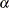value for leverage-point detection. The respective leverage cutoff value equals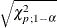(or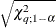if projection is applied in the generalized MCD algorithm). By default,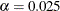.

H=n
QUANTILE=n

specifies the quantile to be minimized for the MCD algorithm that is used for the leverage-point analysis. By default, H=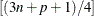, where n is the number of observations and p is the number of independent variables, excluding the intercept.

MCDALPHA=alpha-value

specifies the MCD cutoffvalue for the final MCD reweighting step. The respective MCD cutoff value equals(orif projection is applied in the generalized MCD algorithm). By default,.

MCDCUTOFF=value
MCDCUT=value

specifies the MCD cutoff value for the final MCD reweighting step. For more information, see the section Mahalanobis Distance versus Robust Distance and Rousseeuw and Van Driessen (1999). You can also specify the cutoff value by using the MCDALPHA= option.

MCDINFO

requests that detailed information about the MCD covariance estimate be displayed, including the low-dimensional structure, the breakdown value, the MCD center, and the MCD covariance itself. The option outputs the ODS tables of the MCD profile, MCD center, MCD covariance, and MCD correlation.

OPC | OFFPLANECOEF

requests the ODS table of the coefficients for MCD-dropped components, when projection is applied in the generalized MCD algorithm. The OFFPLANECOEF option is ignored for the regular MCD algorithm.

PROJECTIONALPHA=alpha-value
PALPHA=alpha-value

specifies the projection cutoffvalue to be used to judge whether an observation is on or off the low-dimensional hyperplane that is identified by the generalized MCD algorithm. The respective projection cutoff value equals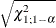. By default,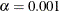.

PROJECTIONCUTOFF=value
PCUTOFF=value

specifies the projection cutoff value to be used to judge whether an observation is on or off the low-dimensional hyperplane identified by the projected MCD algorithm. For more information, see the section Mahalanobis Distance versus Robust Distance and Rousseeuw and Van Driessen (1999). You can also specify the projection cutoff value by using the PALPHA= option.

PROJECTIONTOLERANCE=value
PTOL=value

specifies the projection tolerance value for the low-dimensional structure detection. For more information, see the section Leverage-Point and Outlier Detection.

NOGOODFIT

suppresses the computation of goodness-of-fit statistics.

NOINT

specifies no-intercept regression.

SINGULAR=value

specifies the tolerance for testing singularity of the information matrix and the crossproducts matrix for the initial least squares estimates. By default, SINGULAR=1E–12.